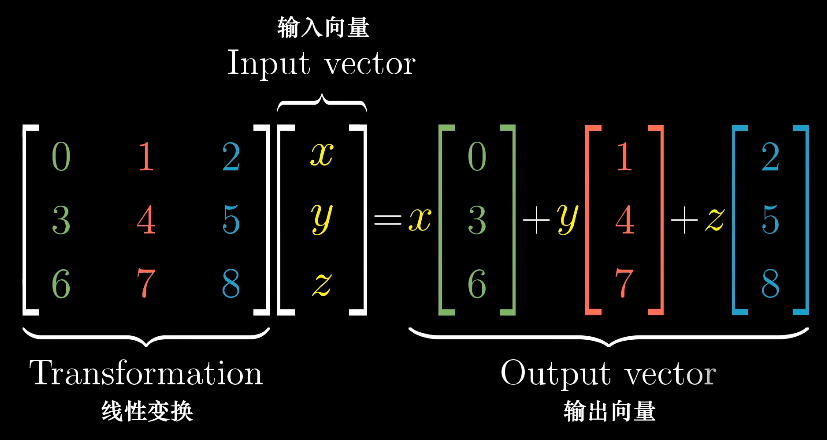### 线性基

albus就是要第一个出场就是求子集异或和中某个数的排名，直接$2^{n-cnt}*findrk+1$即可

# 真·线性代数

### 普适的代价是抽象​ 代数理解：相应坐标配对乘后求和，显然满足交换律

​ 几何理解：A在B上的带符号投影乘B的长度，即$A \cdot B=|A||B|cos \theta$，不显然满足交换律，如何用直观的方式理解？先考虑两个向量的模一样时显然成立，然后你把某个伸缩依然成立

​ 然后也可以理解为从n维变换到1维，即把某个向量横过来做矩阵乘法（$A \cdot B=A^TB$）### 接下来从代数角度考虑（主要抄自雅礼201903的课件）

n阶方阵：就是大小为$n \times n$ 的矩阵

1. 根据通项公式，行列地位平等，转置不影响行列式
2. 三角矩阵和某些特殊的矩阵的行列式是主对角线元素乘积，因为合法p只有对角线
3. 交换任意两行、列后行列式取反（所以用高斯消元求行列式的话要注意），证明：其实就是证排列交换两个元素后逆序对数的奇偶性必定变化，考虑到交换相邻一定改变，而现在要做奇数次相邻交换，证毕（upd：好像也没必要这么证）。
4. 性质2的推论，两行完全相同下行列式=0；进一步发现，两行成比例的话也=0，因为可以把比例系数放到行列式的外面；于是你发现我们对行列式的几何理解与我们现在的数学推导是吻合的（当然我并不知道怎么相互推导）
5. 可以把某一行拆分成序列A和序列B的和，然后行列式就是两个其他行不变的矩阵的行列式之和。这个比较显然，和性质3放在一起可知，做初等行列变换（带系数叠加去某行）不影响行列式
6. $|A*B|=|A||B|$，证明考虑行与列做点积

$当det(A) \ne 0，x_i=\frac{det(A_i)}{det(A)}，其中A_i表示用c取代A的第i列得到的矩阵$

k阶子式：选k行k列组成的k阶方阵的行列式

$(一堆初等变换矩阵)*A=E，A^{-1}A=E$ ，如果你认可A的逆矩阵唯一（几何意义不难理解），那么求矩阵的逆就是将A高斯消元成E，然后按原顺序将过程中所做的初等变换在E上做一次，洛谷板子

n元齐次方程组： $Ax=0$ 有非0解的充要条件是$r(A)<n$；且不难发现他的解集是一个向量空间

$P^{-1}AP=diag(\lambda_1,\lambda_2,..\lambda_n),AP=Pdiag(\lambda_1,\lambda_2,..\lambda_n),AP_i=\lambda P_i$ ，即P的每个列向量都是A的特征向量，又因P可逆，故A可对角化的充要条件是有n个线性无关的特征向量（而任意n阶方阵最多n个）。

### 常系数线性齐次递推

Hamilton-Cayley theorem：$f(A)=0$ （A的特征多项式），并不是什么前沿科技其实，dzy的证明

$$A-\lambda I= \left[ \begin{matrix} a_1-\lambda & a_2 &…& a_n \\ 1 & -\lambda &…& 0 \\ 0 & 1 & -\lambda & 0 \\ 0 & 0 & 1 & -\lambda \end{matrix} \right]， g= \left[ \begin{matrix} a_{n-1} \ a_{n-2} \ … \ a_0 \ \end{matrix} \right] \\ f(\lambda)=det(A-\lambda I)=(-\lambda)^n+\sum_{j=1}^n a_j (-1)^{j+1} (-\lambda)^{n-j}=(-\lambda)^n+(-1)^{n+1}\sum_{j=1}^n a_j \lambda^{n-j} \\ f(A)=0,A^n=\sum_{j=1}^n a_jA^{n-j} ，可见任何 A^k都可以被表示为 A^{0 \sim n-1}的线性组合 \\ ans=(A^eG)[n,1]，考虑表示为 A^{0 \sim n-1}的线性组合即 \sum_{i=0}^{n-1}q_i(A^iG)[n,1]=\sum_{i=0}^{n-1}q_ih_i，h为所求序列 \\ 考虑这个q以及f(A)=0，你发现我们其实就是在做矩阵多项式模，A^e=f(A)p(A)+q(A) \\ 考虑怎么用n相关的复杂度计算多项式模，你发现我们左边是个幂，所以我们不妨用快速幂来搞 \\ A^{2k}=A^k \times A^k(\% f(A))，暴力做（用解线性组合做取模）就是O(m^2logn)，写全家桶就是O(mlogmlogn)$$

### Matrix-Tree定理

Matrix-Tree定理：无向简单图的生成树个数=C的任意一个代数余子式值；证明1证明-lca

### best定理

$从st出发的个数\ = \ cnt*deg_{st} \ \prod (deg_x-1)!$（如果边的环同构算一种方案，就不要乘$deg_{st}$）

### 循环矩阵

$$\left{ \begin{matrix} x_0&x_1&x_2&…&x_{n-1} \\ x_{n-1}&x_0&x_1&…&x_{n-2} \\ x_{n-2}&x_{n-1}&x_0&…&x_{n-3}\\ \vdots &\vdots &\vdots &\ddots & \vdots \\ x_1&x_2&x_3&…&x_0 \end{matrix} \right} ，发现两个循环矩阵的乘积依然是循环矩阵 \\ C_{0,i}=\sum_{j=0}^{n-1} A_{0,j}*A_{j,i}， 根据循环可知 C_{(i+j) \bmod n}=\sum_{i=0}^{n-1} A_i*A_j，FFT优化卷积O(nlognlogk)，exp的话假装O(nlogn)$$